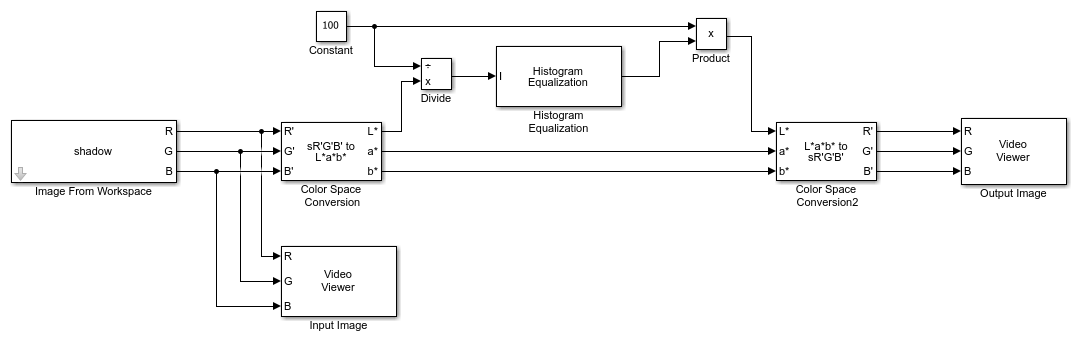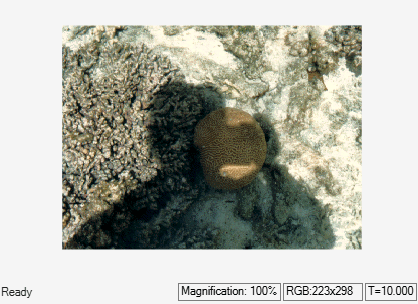# Enhance Contrast of Color Image Using Histogram Equalization

This example shows how to enhance the contrast of a color image using the Histogram Equalization block.

### Example Model

```modelname = 'ex_blkHistEqColor.slx'; open_system(modelname) ```### Initialize Parameters

The model uses the initialization function callback `InitFcn` to initialize the input parameters. To configure the `InitFcn`, on the Modeling tab, click Model Settings and select Model Properties. In the Model Properties dialog box, click the Callbacks tab and select `InitFcn` from the list.

The `InitFcn` of this model executes the following code to read an indexed image and convert it to an RGB image.

```[X,map] = imread('shadow.tif'); shadow = ind2rgb(X,map); ```

This model reads the input image from workspace using the Image From Workspace block with these block parameters:

• Value: `shadow`

• Image signal: `Separate color signals`

### Enhance Contrast of Image

The model extracts luminance information from the color image by converting it from the RGB color space to L*a*b* color space, using the Color Space Conversion block with these parameters:

• Conversion: `sR'G'B' to L*a*b*`

• Image signal: `Separate color signals`

The values of the luminance parameter `L*` range from 0 to 100. The Histogram Equalization block expects floating point input values in the range from [0, 1]. The model normalizes the luminance values of the image by using a Constant block with the Constant value parameter set to `100` and a Divide block with default parameters.

To enhance the contrast of the image, the model uses a Histogram Equalization block with default parameters. The block transforms the normalized luminance values in the input color image such that the histogram of the output image is approximately uniform.

The model uses a Product block with default parameters and the existing Constant block to scale the luminance of the histogram-equalized output image to a range of 0 to 100. The model then converts the output image from the L*a*b* color space to the RGB color space using a Color Space Conversion block with these parameters:

• Conversion: `L*a*b* to sR'G'B'`

• Image signal: `Separate color signals`

### Simulate and Display Results

Run the model and verify the change in contrast from input image to the output image. For comparison, the model displays the input image and the output image using Video Viewer blocks. The Image signal parameter of each Video Viewer block is set to `Separate Color Signals` .

```sim(modelname); ```